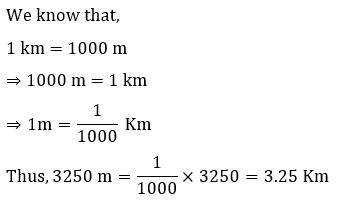# NCERT Solutions for Class 6 Science Chapter 10 Motion and Measurements of Distances

Question 1. Give two examples each, of modes of transport used on land, water and air.

Answer:- Modes of transport used on land, water and air are following:-

1. Land – Bus and Car
2. Water – Ship and Boat
3. Air – Helicopter and Aeroplane.

Question 2. Fill in the blanks:

• (i) One metre is ______________ cm.
• (ii) Five kilometre is ______________ m.
• (iii)Motion of a child on a swing is ______________.
• (iv)Motion of the needle of a sewing machine is ______________.
• (v) Motion of wheel of a bicycle is______________.

• (i) One metre is 100 cm.
• (ii) Five kilometre is 5000 m.
• (iii)Motion of a child on a swing is periodic
• (iv)Motion of the needle of a sewing machine is periodic
• (v) Motion of wheel of a bicycle is circular.

Question 3. Why can a pace or a footstep not be used as a standard unit of length?

Answer:- A pace or a footstep varies in size from person to person because of this they are not be used as a standard unit of length.

Question 4. Arrange the following lengths in their increasing magnitude:

1 metre, 1 centimetre, 1 kilometre,1 millimetre.

Answer:- 1 millimetre, 1 centimetre, 1 metre, 1 kilometre

Question 5. The height of a person is 1.65 m. Express it into cm and mm.

Answer:- We know that,

(a) 1.65 m

1 metre = 100 cm

1.65 m = 1.65 x 100 cm

165 cm

(b) 1 metre = 1000 mm

1.65 m = 1.65 x 1000 mm

1650 cm

Question 6. The distance between Radha’s home and her school is 3250 m. Express this distance into km.Question 7. While measuring the length of a knitting needle, the reading of the scale at one end is 3.0 cm and at the other end is 33.1 cm. What is the length of the needle?

Answer:-Length of the needle = 33.1 cm – 3.0 cm = 30.1 cm.

Question 8. Write the similarities and differences between the motion of a bicycle and a ceiling fan that has been switched on.

Answer:- Similarities – Both show circular motion

Differences :- Bicycle move in rectilinear motion whereas the fan does not move in rectilinear motion.

Question 9. Why could you not use an elastic measuring tape to measure distance? What would be some of the problems you would meet in telling someone about a distance you measured with an elastic tape?

Answer:- An elastic measuring tape reduces in size when released after stretched. So it will not give accurate measurement. It would be difficult to tell someone how much it was streched while measuring.

Question 10. Give two examples of periodic motion.

Answer:- Two examples of periodic motion are:-

1. a) Motion of swing
2. b) Pendulum

Click on the Chapter’s Link to get PDF of all Chapters of  NCERT of Science Class 6